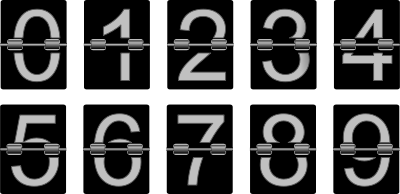# Sequences using JavaScript ArrayGenerating a sequence is a common programming task. This is rather easy to achieve using a straightforward loop. With JavaScript however, there exists a more functional variant using the powerful `Array` object. This permits the generation of all kind of sequences, from `A..Z` to a list of prime numbers, without any loops at all.

Supposed you are about to create a list of numbers `1, 2, 3` and place it in an array, one possible loop-based implementation is:

``````var result = [];
for (var i = 1; i != 4; ++i) result.push(i)
console.log(result);  // [1, 2, 3]
``````

Obviously, tweaking the code can yield a different kind of sequence. For example, a sequence of Latin alphabets is a matter of converting each number into the right letter:

``````var list = '';
for (var i = ; i != 26; ++i) list += String.fromCharCode(i + 65);
console.log(list);   // 'ABCDEFGHIJKLMNOPQRSTUVWXYZ'
``````

Using loops is nice, but can we get the same result sans loops? Fortunately, JavaScript is quite capable of doing it. We just need to rely on its built-in object `Array`. In the following explanation, all the sections mentioned refer to the Standard ECMA-262, the official ECMAScript Language Specification edition 5.1.

#### Earth

First of all, we need to create an array which has the specified amount of elements. For that `1,2,3` example, we need a 3-element array. Fortunately, this is trivial:

``````Array(3);
``````

Note that there is no need to use new for object construction, this is explained in Section 15.4.1:

When Array is called as a function rather than as a constructor, it creates and initialises a new Array object.

`Array(3)` creates an array with the length of 3, it is the same as `[,,,]`. The resulting array however has holes in it. In fact, although it has 3 elements, those elements do not exist. Holes like this are not so obvious if you try to peek at the array contents. Compare the two lines:

``````Array(3).join('-');                // "--"
[null,undefined,null].join('-');   // "--"
``````

We can verify the existence of an array element just like checking for a property in a generic JavaScript object, using the `in` operator (Section 11.8.7):

``````in Array(3);     // false
1 in Array(3);   // false
2 in Array(3);   // false
2 in [,,9];      // true
``````

As pointed by Axel Rauschmayer, holes inside an array are also detected with `forEach`.

#### Water

How to fill those holes? A trick discovered by many seasoned JavaScript developers (see e.g. Brandon Benvie’s post on es-discuss) is to use `Array.apply`. Instead of some empty elements, now we have `undefined` to replace them:

``````Array(3);                  // [,,,]
Array.apply(undefined, Array(3));  // [undefined, undefined, undefined]
``````

To really understand this trick, recall how `Function.prototype.apply` works (Section 15.3.4.3), particularly in the Step 8, transforming an array into an argument list for the function, called spreading. No wonder this approach is quite often used to find the minimum or maximum value in an array. In the following fragment, the two lines are identical:

``````Math.max(14, 3, 77);                 // 77
Math.max.apply(Math, [14, 3, 77]);   // 77
``````

When `apply` receives an array with an empty element, it gets converted into `undefined` and thereby eliminates any holes in the array. If we combined it with `Array` construction, the end effect is constructing a new array with the spread.

``````Array.apply(undefined, [1,,3]);  // is the same as
Array(1, undefined, 3);
``````

#### Air

Now that we have an array with the right number of elements, how do fill it with the right sequence? `Array.prototype.map` to the rescue! Section 15.4.4.19 shows that:

map calls callbackfn once for each element in the array, in ascending order, and constructs a new Array from the results.

Further down, we also observe that:

callbackfn should be a function that accepts three arguments. callbackfn is called with three arguments: the value of the element, the index of the element, and the object being traversed.

The second argument, the index of the element, is the key to our solution:

``````Array.apply(undefined, Array(3)).map(function (x, y) { return y + 1; });  // [1, 2, 3]
``````

And if the sequence is about the squares of the first few integers:

``````Array.apply(undefined, Array(3)).map(function (x, y) { return (y + 1) * (y + 1); });
``````

Finally, for the English alphabets ‘ABCDEFGHIJKLMNOPQRSTUVWXYZ’:

``````Array.apply(undefined, Array(26)).map(function(x,y) {
return String.fromCharCode(y + 65);
}).join('');
``````

For alternatives to using `map`, see also Brandon Benvie’s usage of Function.call with Number or Ben Alman’s solution with Object.keys.

#### Fire

With the new array comprehension feature of the forthcoming ECMAScript 6, the above one-liners can be slightly tweaked. For example, the alphabets sequence might be written as (note the use of arrow function):

``````[for (i of Array.apply(undefined, Array(26)).map((x, y) => y))
String.fromCharCode(65 + i)].join('');
``````

Here the conversion from each number to the corresponding letter is carried out outside the `map` callback. This makes the code easier to digest, it resembles a proper composition: generate the sequence first and do another step to transform the sequence. For details, check out my previous blog post (with tons of examples) on ECMAScript 6 and Array Comprehension.

Loops are overrated, aren’t they?

Addendum: As a follow-up, see also how you can use the same approach to generate a list of prime numbers, compute the factorial, and produce the Fibonacci series in the new blog post: Prime Numbers, Factorial, and Fibonacci Series with JavaScript Array.

Update: If you work in an environment that supports ES2015 standard (often informally known also as ES6), you can avoid the hassle of using `Array.apply` since it is easier to use Array#fill. Instead of:

``````Array.apply(undefined, Array(3)).map((x, y) => y); // [0, 1, 2]
``````

simply write:

``````Array(3).fill(0).map((x, y) => y);    // [0, 1, 2]
``````

`````` [...Array(3).keys()];    // [0, 1, 2]